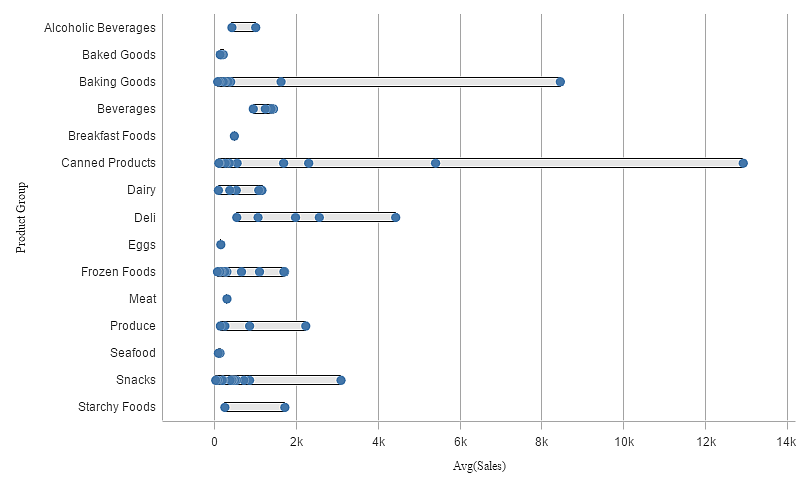# Distribution plot

The distribution plot is suitable for comparing range and distribution for groups of numerical data. Data is plotted as value points along an axis.

You can choose to display only the value points to see the distribution of values, a bounding box to see the range of values, or a combination of both as shown here:## When to use it

The distribution plot is suitable for comparing range and distribution for groups of numerical data.

The distribution plot visualizes the distribution of data.

The distribution plot is not relevant for detailed analysis of the data as it deals with a summary of the data distribution.

## Creating a distribution plot

You can create a distribution plot on the sheet you are editing.

In a distribution plot you need to use one or two dimensions, and one measure. If you use a single dimension you will receive a single line visualization. If you use two dimensions, you will get one line for each value of the second, or outer, dimension.

1. In a sheet, clickto add a new visualization.
2. Under Visualization, select Distribution plot.

This is the inner dimension, which defines the value points.

4. Optionally, add a second dimension.

This is the outer dimension, which defines the groups of value points shown on the dimension axis.

When you have created the distribution plot, you may want to adjust its appearance and other settings in the properties panel.